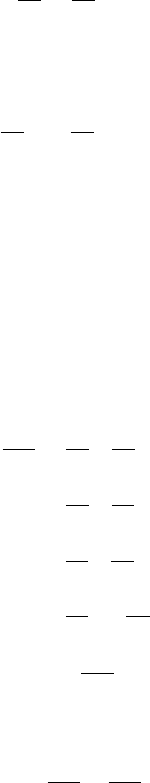1Analytic functions

IB Complex Methods1.3 Harmonic functions
This is the last easy section of the course.
Definition
(Harmonic conjugates)
.
Two functions
u, v
satisfying the Cauchy-
Riemann equations are called harmonic conjugates.
If we know one, then we can find the other up to a constant. For example, if
u(x, y) = x
2
y
2
, then v must satisfy
v
y
=
u
x
= 2x.
So we must have
v
= 2
xy
+
g
(
x
) for some function
g
(
x
). The other Cauchy-
Riemann equation gives
2y =
u
y
=
v
x
= 2y g
0
(x).
This tells us
g
0
(
x
) = 0. So
g
must be a genuine constant, say
α
. The corresponding
analytic function whose real part is u is therefore
f(z) = x
2
y
2
+ 2ixy + = (x + iy)
2
+ = z
2
+ iα.
Note that in an exam, if we were asked to find the analytic function
f
with real
part
u
(where
u
is given), then we must express it in terms of
z
, and not
x
and
y, or else it is not clear this is indeed analytic.
On the other hand, if we are given that
f
(
z
) =
u
+
iv
is analytic, then we
can compute
2
u
x
2
=
x
u
x
=
x
v
y
=
y
v
x
=
y
u
y
=
2
u
y
2
.
So u satisfies Laplace’s equation in two dimensions, i.e.
2
u =
2
u
x
2
+
2
u
y
2
= 0.
Similarly, so does v.
Definition
(Harmonic function)
.
A function satisfying Laplace’s equation equa-
tion in an open set is said to be harmonic.
Thus we have shown the following:
Proposition.
The real and imaginary parts of any analytic function are har-
monic.